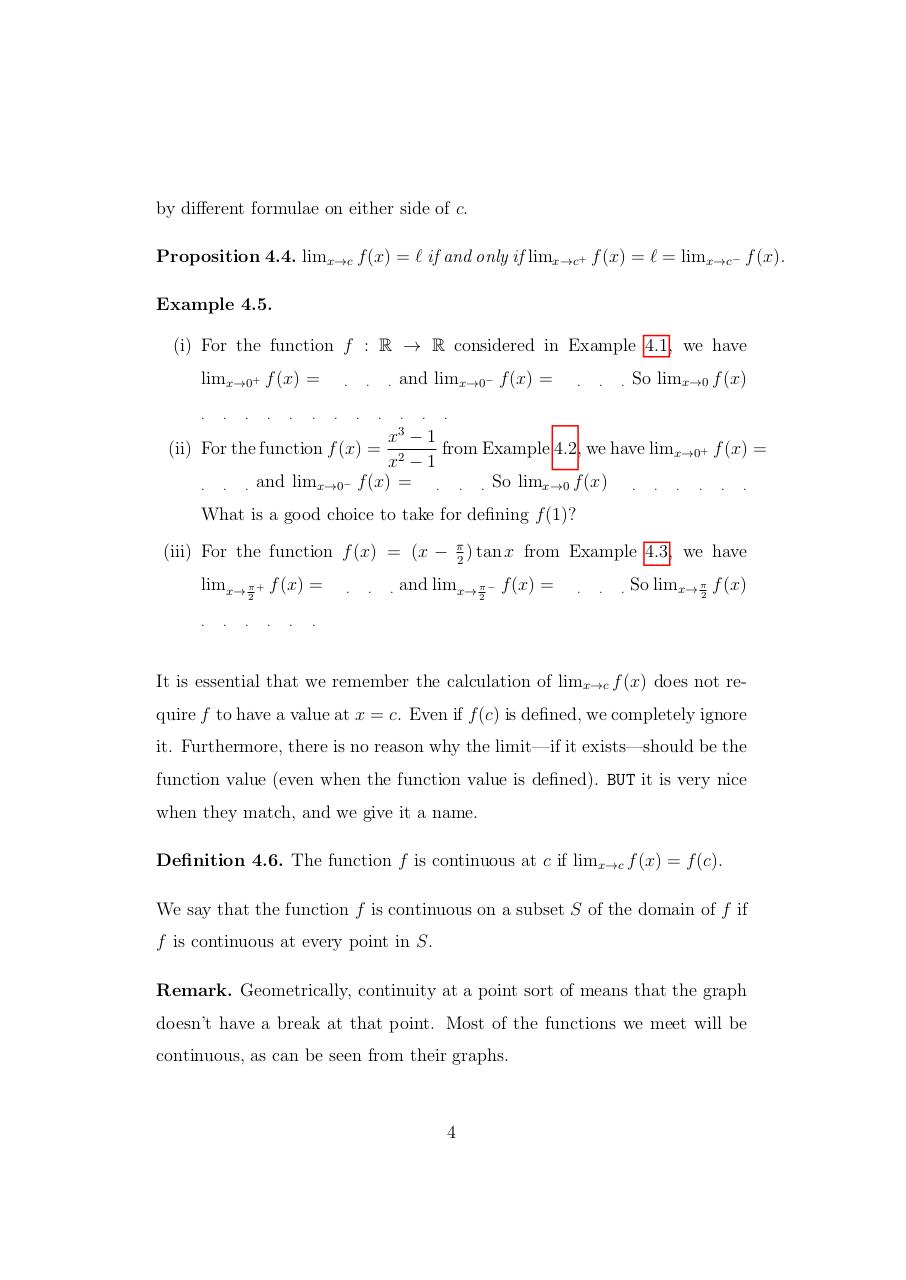# Limits.pdfPage 1 2 3 4 5 6 7 8 9 10 11 12

#### Text preview

by different formulae on either side of c.
Proposition 4.4. limx→c f (x) = ` if and only if limx→c+ f (x) = ` = limx→c− f (x).
Example 4.5.
(i) For the function f : R → R considered in Example 4.1, we have
limx→0+ f (x) =
.

.

.

.

.

.

.
.

.
.

.
.

and limx→0− f (x) =
.
3

.

.

.

.

So limx→0 f (x)

.

x −1
from Example 4.2, we have limx→0+ f (x) =
x2 − 1
and limx→0− f (x) = . . . So limx→0 f (x) . . . . . .

(ii) For the function f (x) =
.

.

.

What is a good choice to take for defining f (1)?
(iii) For the function f (x) = (x − π2 ) tan x from Example 4.3, we have
limx→ π + f (x) =
2

.

.

.

.

.

.

.

.

and limx→ π − f (x) =
2

.

.

.

So limx→ π2 f (x)

.

It is essential that we remember the calculation of limx→c f (x) does not require f to have a value at x = c. Even if f (c) is defined, we completely ignore
it. Furthermore, there is no reason why the limit—if it exists—should be the
function value (even when the function value is defined). BUT it is very nice
when they match, and we give it a name.
Definition 4.6. The function f is continuous at c if limx→c f (x) = f (c).
We say that the function f is continuous on a subset S of the domain of f if
f is continuous at every point in S.
Remark. Geometrically, continuity at a point sort of means that the graph
doesn’t have a break at that point. Most of the functions we meet will be
continuous, as can be seen from their graphs.

4